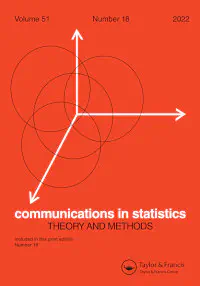# Modeling Sums of Exchangeable Binary Variables### Abstract

We introduce a new model for sums of exchangeable binary random variables. The proposed distribution is an approximation to the exact distributional form, and relies on the theory of completely monotone functions and the Laplace transform of a gamma distribution function. Using Monte Carlo methods, we show that this new model compares favorably to the beta-binomial model with respect to estimating the success probability of the Bernoulli trials and the correlation between any two variables in the exchangeable set. We apply the new methodology to two classic data sets and the results are summarized.

Publication
In Communications in Statistics – Theory and Methods